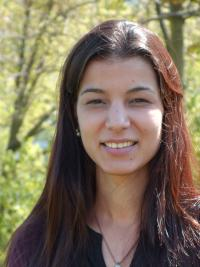# Dr. Denise Moreira de GodoyDr. Denise Moreira de Godoy

postdoctoral research fellow
field of research: ALICE

Room 201
Tel.: +49 251 83-34993
E-Mail: moreirad [AT] uni-muenster.de
Consultation hours: by appointment

• ### Publications

• Acharya S, others. . ‘Neutral pion and $η$ meson production in p-Pb collisions at $\sqrt{s_\mathrm{NN}}} = 5.02$ TeV}.’ Eur. Phys. J. C78, No. 8: 624. doi: 10.1140/epjc/s10052-018-6013-8.
• Acharya S, others. . ‘Constraints on jet quenching in p-Pb collisions at $\mathbf{\sqrt{s_{NN}}}}}$ = 5.02 TeV measured by the event-activity dependence of semi-inclusive hadron-jet distributions}.’ Phys. Lett. B783: 95-113. doi: 10.1016/j.physletb.2018.05.059.
• Acharya S, others. . ‘$\Lambda_\mathrm{ c}^+$ production in pp collisions at $\sqrts = 7$ TeV and in p-Pb collisions at $\sqrt{s_\mathrm{ NN}}} = 5.02$ TeV}}}.’ JHEP 04: 108. doi: 10.1007/JHEP04(2018)108.
• Acharya S, others. . ‘Measurement of Z$^0$-boson production at large rapidities in Pb-Pb collisions at $\sqrt{s_\mathrm{ NN}}}=5.02$ TeV}.’ Phys. Lett. B780: 372-383. doi: 10.1016/j.physletb.2018.03.010.
• Acharya S, others. . ‘First measurement of $\Xi_\mathrm{ c}^0$ production in pp collisions at $\mathbf{\sqrts}}$ = 7 TeV}}.’ Phys. Lett. B781: 8-19. doi: 10.1016/j.physletb.2018.03.061.
• Acharya S, others. . ‘Production of $^{4}$He and $^{4}øverline{\textrm{He}}}$ in Pb-Pb collisions at $\sqrt{s_{\mathrm{NN}}}}}$ = 2.76 TeV at the LHC}}}}.’ Nucl. Phys. A971: 1-20. doi: 10.1016/j.nuclphysa.2017.12.004.
• Acharya S, others. . ‘Longitudinal asymmetry and its effect on pseudorapidity distributions in Pb-Pb collisions at $\sqrt{s_{NN}}}$ = 2.76 TeV}.’ Phys. Lett. B781: 20-32. doi: 10.1016/j.physletb.2018.03.051.
• Acharya S, others. . ‘Search for collectivity with azimuthal J/$ψ$-hadron correlations in high multiplicity p-Pb collisions at $\sqrt{s_\mathrm{ NN}}}$ = 5.02 and 8.16 TeV}.’ Phys. Lett. B780: 7-20. doi: 10.1016/j.physletb.2018.02.039.
• Acharya S, others. . ‘Production of deuterons, tritons, $^{3}$He nuclei and their antinuclei in pp collisions at $\mathbf{\sqrt{{\textit s}}}}}$ = 0.9, 2.76 and 7 TeV}}.’ Phys. Rev. C97, No. 2: 024615. doi: 10.1103/PhysRevC.97.024615.
• Acharya S, others. . ‘$π ^{0}$ and $η$ meson production in proton-proton collisions at $\sqrts=8$ TeV}}.’ Eur. Phys. J. C78, No. 3: 263. doi: 10.1140/epjc/s10052-018-5612-8.
• Acharya S, others. . ‘$D$-meson azimuthal anisotropy in midcentral Pb-Pb collisions at $\mathbf{\sqrt{s_\mathrm{ NN}}}=5.02}}$ TeV}.’ Phys. Rev. Lett. 120, No. 10: 102301. doi: 10.1103/PhysRevLett.120.102301.
• Acharya S, others. . ‘Systematic studies of correlations between different order flow harmonics in Pb-Pb collisions at $\sqrt{s_\mathrm{ NN}}}$ = 2.76 TeV}.’ Phys. Rev. C97, No. 2: 024906. doi: 10.1103/PhysRevC.97.024906.
• Acharya S, others. . ‘The ALICE Transition Radiation Detector: construction, operation, and performance.’ Nucl. Instrum. Meth. A881: 88-127. doi: 10.1016/j.nima.2017.09.028.
• Acharya S, others. . ‘Constraining the magnitude of the Chiral Magnetic Effect with Event Shape Engineering in Pb-Pb collisions at $\sqrt{s_\mathrm{NN}}}$ = 2.76 TeV}.’ Phys. Lett. B777: 151-162. doi: 10.1016/j.physletb.2017.12.021.
• Acharya S, others. . ‘First measurement of jet mass in Pb--Pb and p--Pb collisions at the LHC.’ Phys. Lett. B776: 249-264. doi: 10.1016/j.physletb.2017.11.044.
• Moreira De Godoy D, Herrmann F, Klasen M, Klein-Bösing C, Suaide AAP. . ‘Elliptic flow of electrons from beauty-hadron decays extracted from Pb--Pb collision data at $\sqrt{s_\mathrm{ NN}}}$ = 2.76 TeV}.’ Eur. Phys. J. C78, No. 5: 395. doi: 10.1140/epjc/s10052-018-5843-8.